rrrudd

•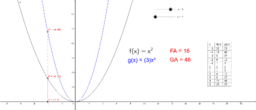Copy of Parameter changes to parent functions - Demonstration

Activity

rrrudd

•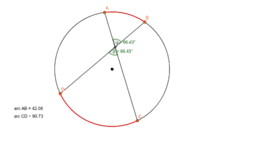Angles and Chords in a Circle

Activity

rrrudd

•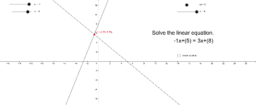Solving linear equations #1

Activity

rrrudd

•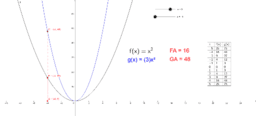Activity

rrrudd

•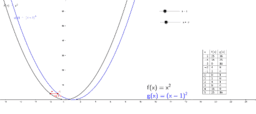Horizontal Translation of a Quadratic Function

Activity

rrrudd

•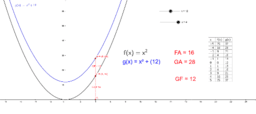Vertical Translation of a Quadratic Function

Activity

rrrudd

•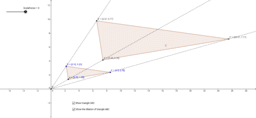Dilations - Triangle

Activity

rrrudd

•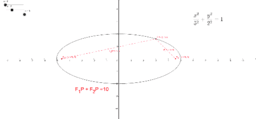Ellipse

Activity

rrrudd

•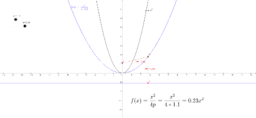Parabola - Focus and Directrix

Activity

rrrudd

•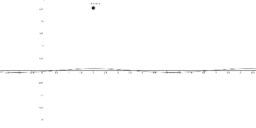Graphical representation for the derivative of y = cosx

Activity

rrrudd

•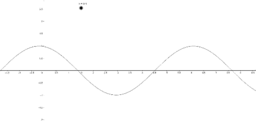Graphical representation for the derivative of y = sinx

Activity

rrrudd

•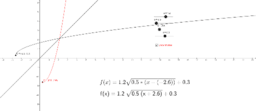Inverse - square root

Activity

rrrudd

•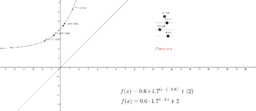Inverse - exponential

Activity

rrrudd

•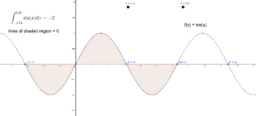Relationship between the definite integral and area

Activity

rrrudd

•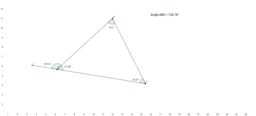Measure of exterior angle of a triangle

Activity

rrrudd

•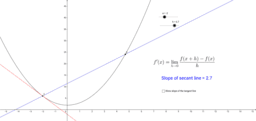Definition of a derivative

Activity

rrrudd

•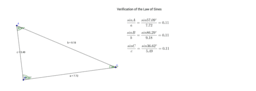Verification of Law of Sines

Activity

rrrudd

•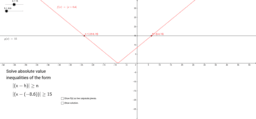Solving absolute value inequalities-greater than or equal to

Activity

rrrudd

•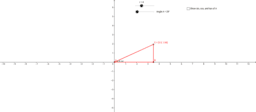Right triangle trigonometry - full revolution

Activity

rrrudd

•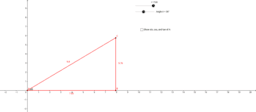Right triangle trigonometry

Activity

rrrudd

•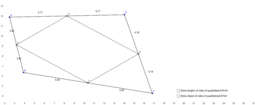Activity

rrrudd

•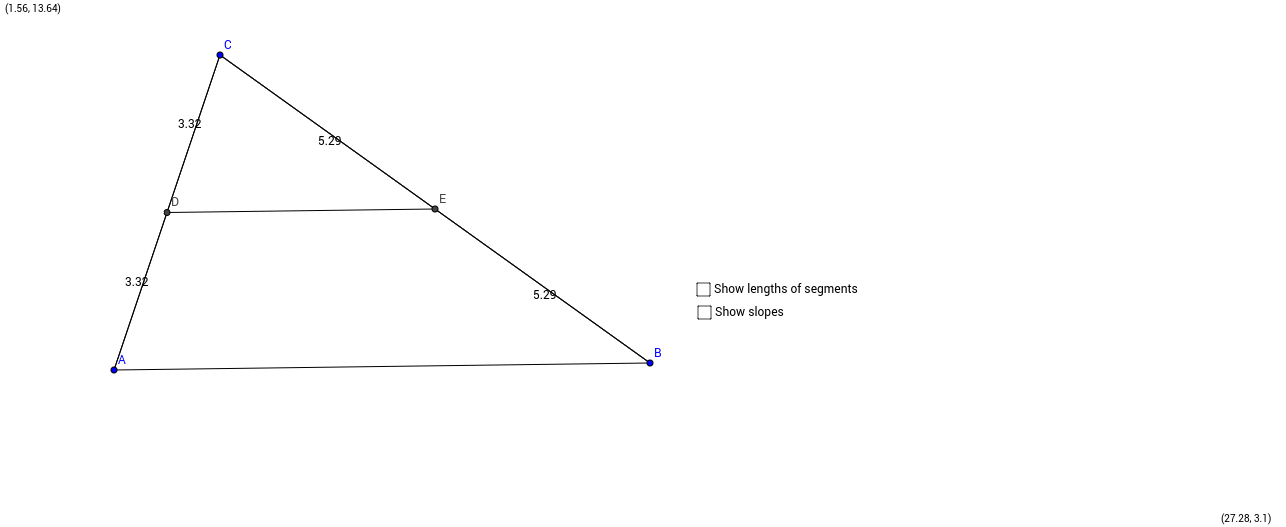Midsegment of a triangle

Activity

rrrudd

•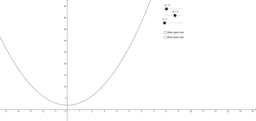Upper sum and lower sum demonstration

Activity

rrrudd

•Activity

rrrudd